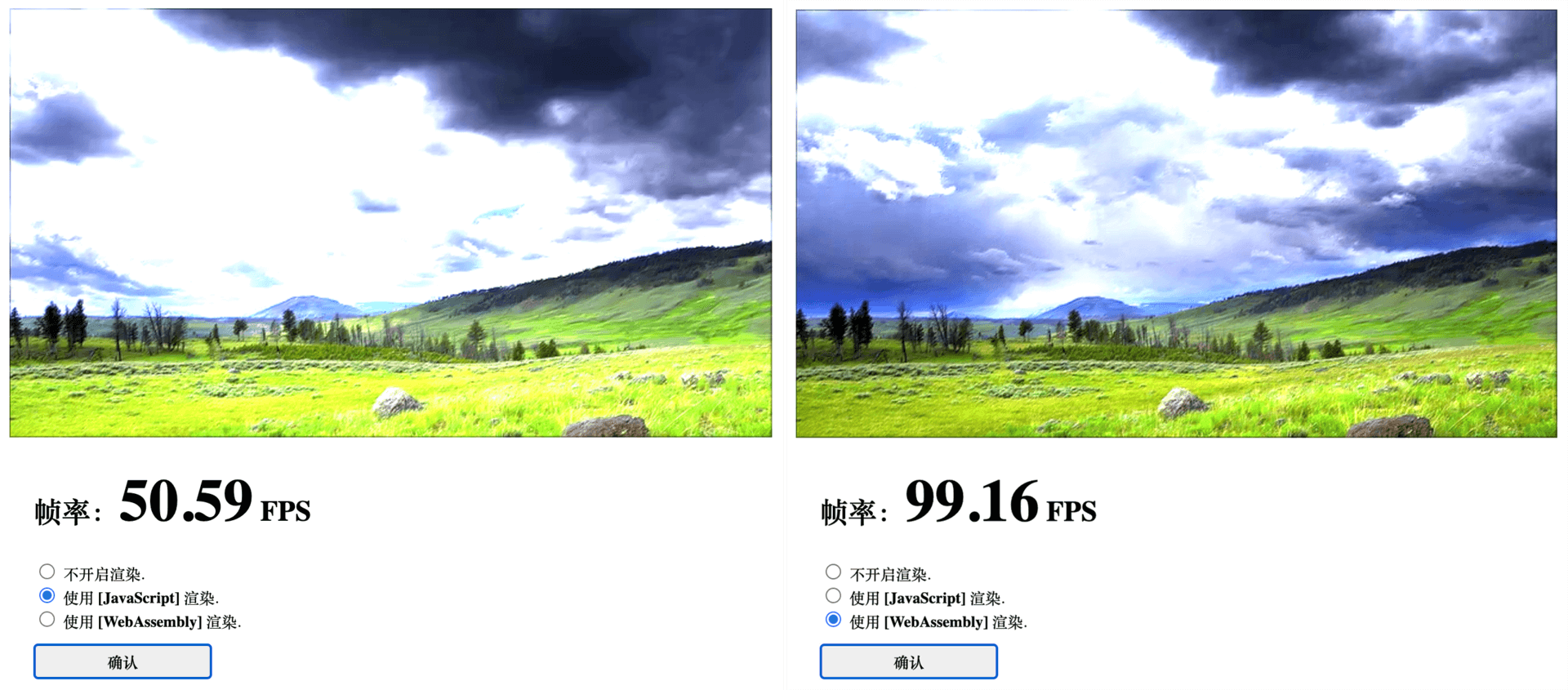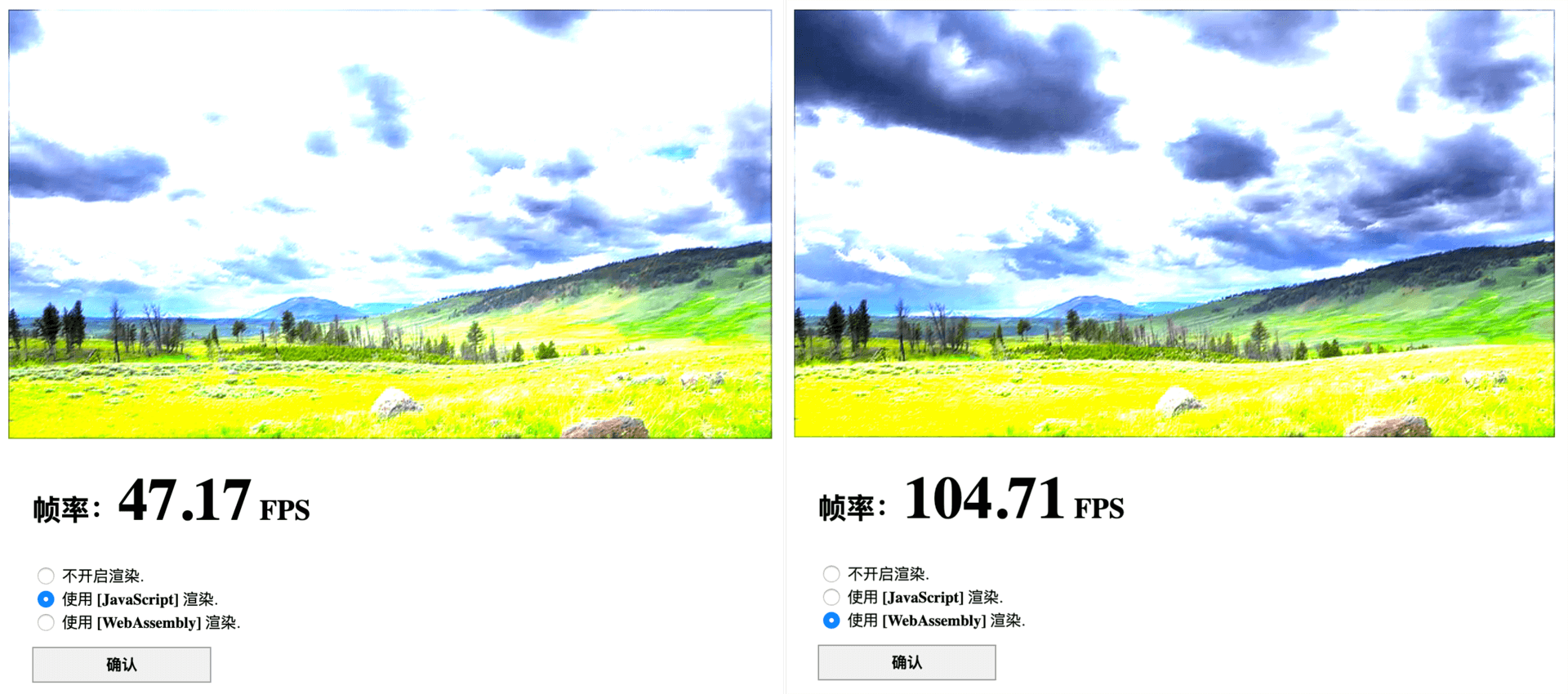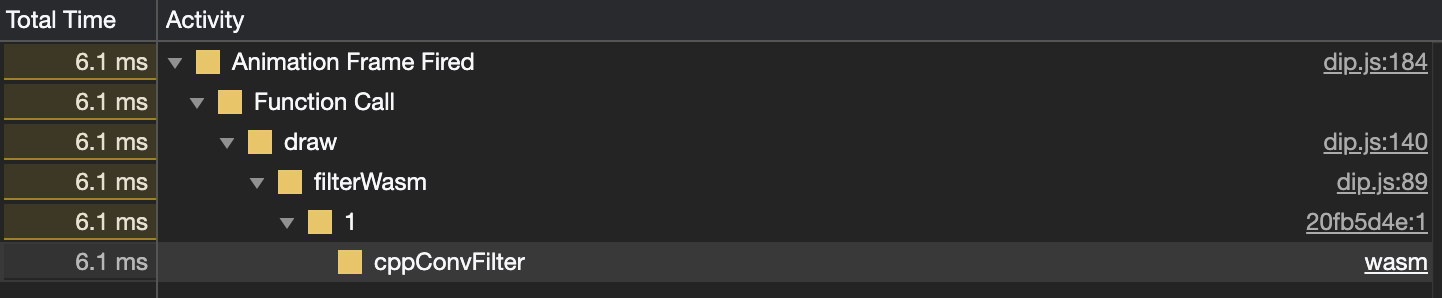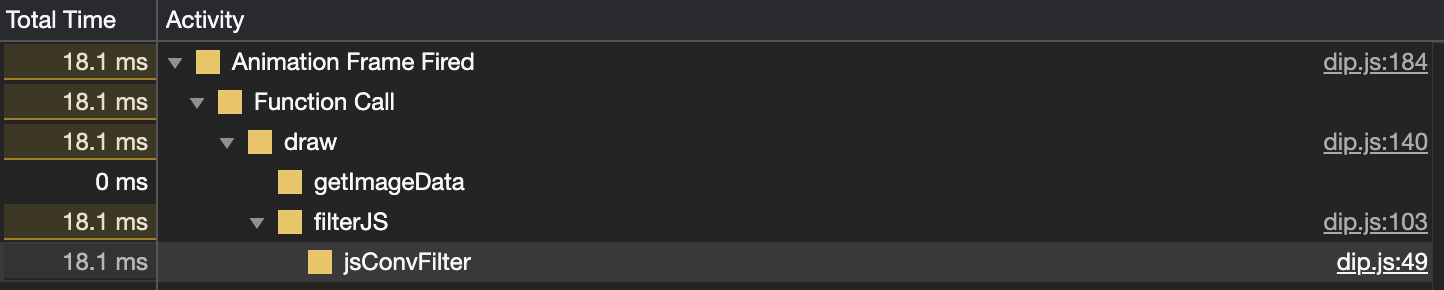17 如何实现一个 WebAssembly 在线多媒体处理应用（三）？

## 编写 C/C++ 函数源码

``````// dip.cc
// 引入必要的头文件；
#include <emscripten.h>
#include <cmath>
// 宏常量定义，表示卷积核矩阵的高和宽；
#define KH 3
#define KW 3
// 声明两个数组，分别用于存放卷积核数据与每一帧对应的像素点数据；
char kernel[KH][KW];
unsigned char data;
// 将被导出的函数，放置在 extern "C" 中防止 Name Mangling；
extern "C" {
// 获取卷积核数组的首地址；
EMSCRIPTEN_KEEPALIVE auto* cppGetkernelPtr() { return kernel; }
// 获取帧像素数组的首地址；
EMSCRIPTEN_KEEPALIVE auto* cppGetDataPtr() { return data; }
// 滤镜函数；
EMSCRIPTEN_KEEPALIVE void cppConvFilter(
int width,
int height,
int divisor) {
const int half = std::floor(KH / 2);
for (int y = half; y < height - half; ++y) {
for (int x = half; x < width - half; ++x) {
int px = (y * width + x) * 4;
int r = 0, g = 0, b = 0;
for (int cy = 0; cy < KH; ++cy) {
for (int cx = 0; cx < KW; ++cx) {
const int cpx = ((y + (cy - half)) * width + (x + (cx - half))) * 4;
r += data[cpx + 0] * kernel[cy][cx];
g += data[cpx + 1] * kernel[cy][cx];
b += data[cpx + 2] * kernel[cy][cx];
}
}
data[px + 0] = ((r / divisor) > 255) ? 255 : ((r / divisor) < 0) ? 0 : r / divisor;
data[px + 1] = ((g / divisor) > 255) ? 255 : ((g / divisor) < 0) ? 0 : g / divisor;
data[px + 2] = ((b / divisor) > 255) ? 255 : ((b / divisor) < 0) ? 0 : b / divisor;
}
}
}
}
``````

`extern "C" {}` 结构中，我们声明了所有需要导出到外部宿主环境（这里为浏览器的 JavaScript 环境）中使用的函数。其中除了 cppConvFilter 函数以外，还有另外的 cppGetkernelPtr 和 cppGetDataPtr 函数。这两个函数主要用来获取先前声明的数组 kernel 与 data 的首地址。通过这种方式，我们便可以在外部的 JavaScript 环境中，向定义在 C/C++ 中的这两个数组结构填充实际的运行时数据了。

## 使用 Emscripten 进行编译

``````emcc dip.cc -s WASM=1 -O3 --no-entry -o dip.wasm
``````

## 整合上下文资源

``````let bytes = await (await fetch('./dip.wasm')).arrayBuffer();
let { instance, module } = await WebAssembly.instantiate(bytes);
let {
cppConvFilter,
cppGetkernelPtr,
cppGetDataPtr,
memory } = instance.exports;
``````

``````// 获取 C/C++ 中存有卷积核矩阵和帧像素数据的数组，在 Wasm 线性内存段中的偏移位置；
const dataOffset = cppGetDataPtr();
const kernOffset = cppGetkernelPtr();
// 扁平化卷积核的二维数组到一位数组，以方便数据的填充；
const flatKernel = kernel.reduce((acc, cur) => acc.concat(cur), []);
// 为 Wasm 模块的线性内存段设置两个用于进行数据操作的视图，分别对应卷积核矩阵和帧像素数据；
let Uint8View = new Uint8Array(memory.buffer);
let Int8View = new Int8Array(memory.buffer);
// 填充卷积核矩阵数据；
Int8View.set(flatKernel, kernOffset);
// 封装的 Wasm 滤镜处理函数；
function filterWASM (pixelData, width, height) {
const arLen = pixelData.length;
// 填充当前帧画面的像素数据；
Uint8View.set(pixelData, dataOffset);
// 调用滤镜处理函数；
cppConvFilter(width, height, 4);
// 返回经过处理的数据；
return Uint8View.subarray(dataOffset, dataOffset + arLen);
}
``````

## 性能对比

• Chrome Version 84.0.4147.89 (Official Build) (64-bit)
• Firefox Version 79.0